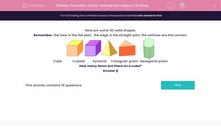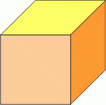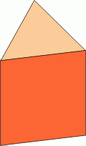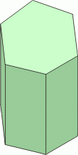# Geometry: Faces, Vertices and Edges of 3D Shapes

In this worksheet, students answer questions on the number of faces, edges and vertices on some 3D shapes.This content is premium and exclusive to EdPlace subscribers.Key stage:  KS 2

Curriculum topic:   Maths and Numerical Reasoning

Curriculum subtopic:   3D Shapes

Difficulty level:#### Worksheet Overview

Here are some 3D solid shapes.

Remember, the face is the flat part,  the edge is the straight part, the vertices are the corners.Cube            Cuboid         Pyramid     Triangular prism  Hexagonal prism

How many faces are there on a cube?